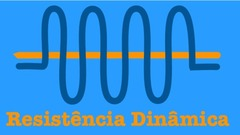RESISTÊNCIA DINÂMICA - Super exponential divergence of the number of periodic points near heterodimensional cyclesDynamical Resistance Day

 Super exponential divergence of the number of periodic points near heterodimensional cycles18 de Setembro | 11h00min | Katsutoshi Shinohara (Hitotsubashi University, Tokyo, Japan)

Title: Super exponential divergence of the number of periodic points near heterodimensional cycles (video)

We say that a diffeomorphism exhibits super exponential divergence of periodic points if (the number of periodic points of period n) times r^n diverges as n \to \infty for every r>0.

I will show that there is a non-empty open set of C^1-diffeomorphisms in which diffeomorphisms in a dense subset exhibit super exponential divergence, which are caused by the bifurcation of heterodimensional cycles.

This is a joint work with Xiaolong Li.